Case Based Questions (MCQ)

Chapter 12 Class 10 Areas related to Circles
Serial order wise
This question is inspired from Ex 12.2, 9 - Chapter 12 Class 10 - Areas Related to Circles

## In a workshop, brooch is made with silver wire  in the form of a circle with diameter 35 mm. The  wire is also used in making 5 diameters which  divide the circle into 10 equal sectors as shown in  the given figure.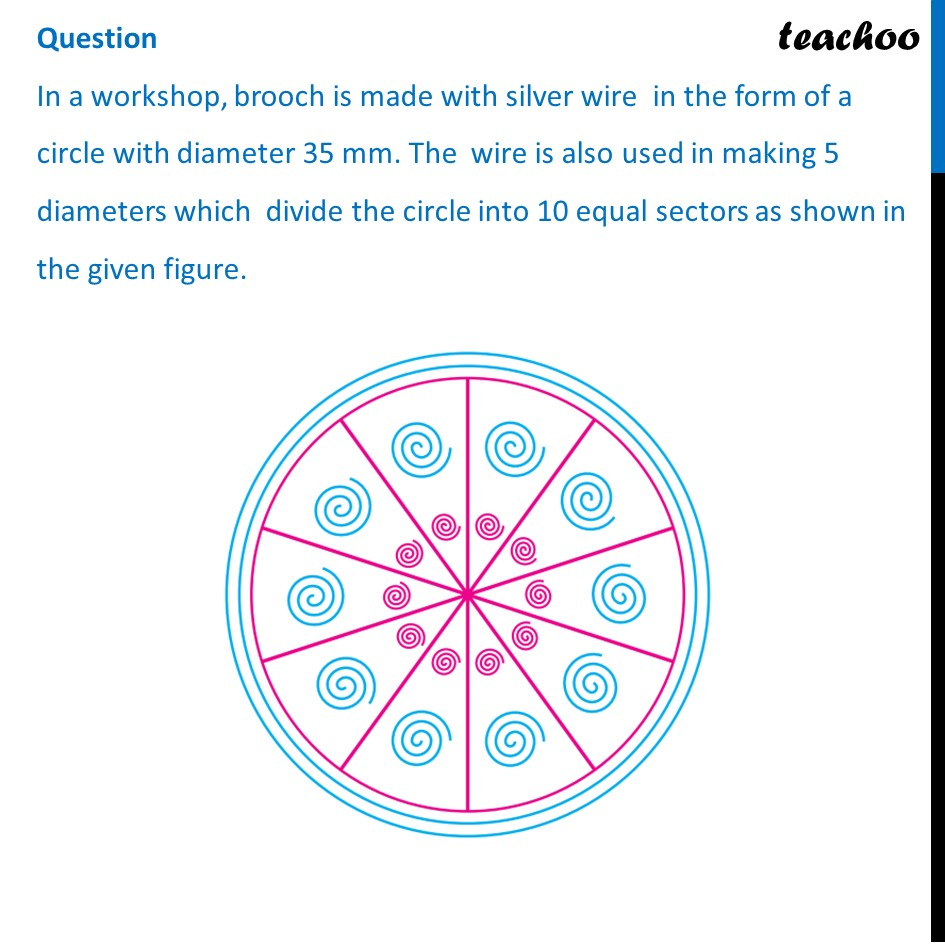## (C) 35 mm             (C) 10 mm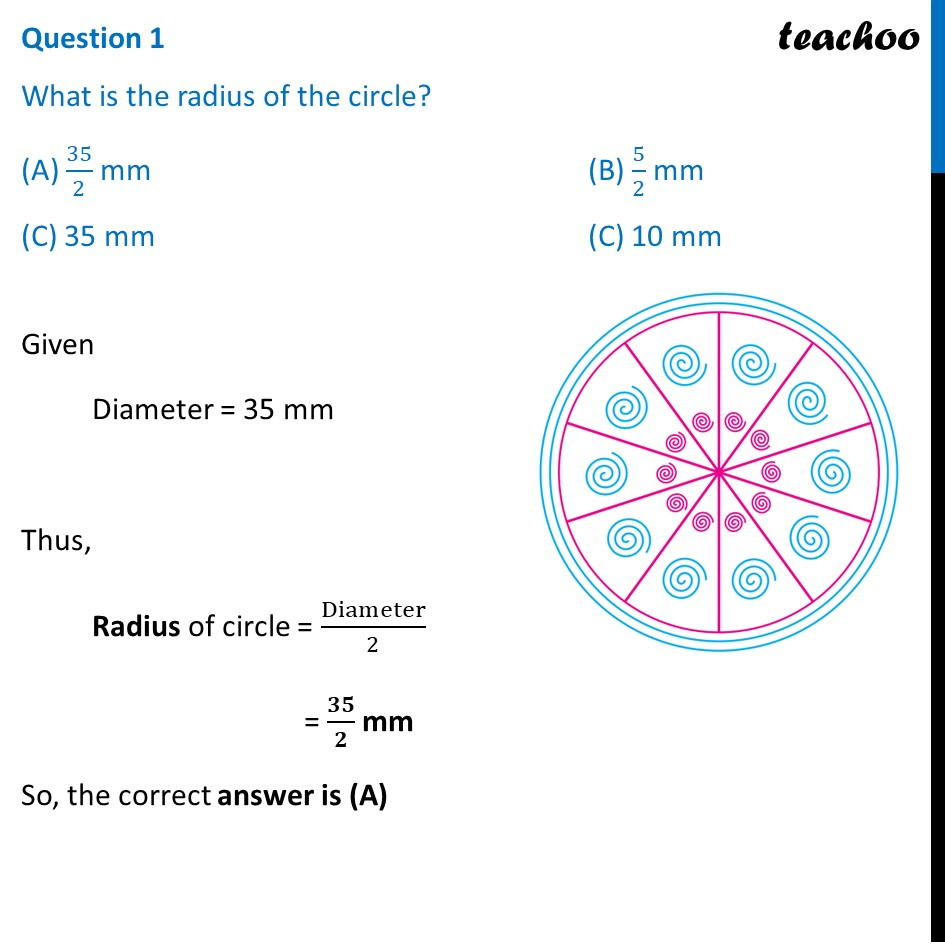## (c) 50 mm                          (d) 10 mm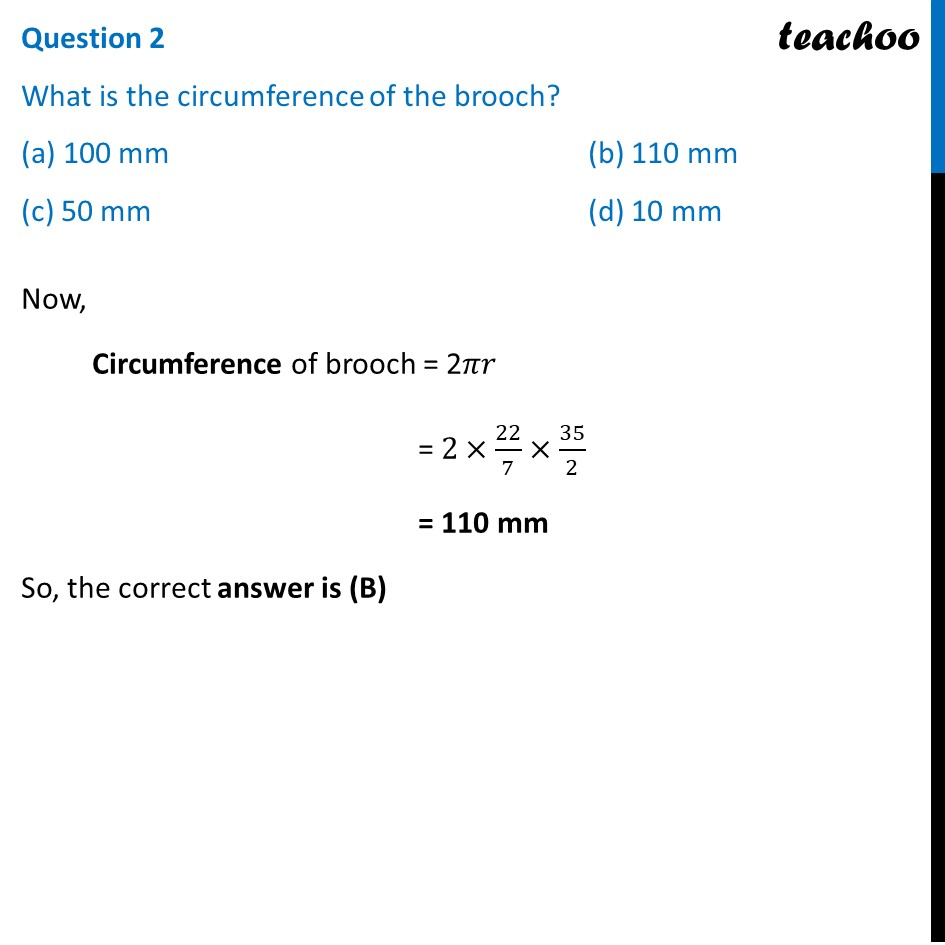## (c) 285 mm                              (d) 852 mm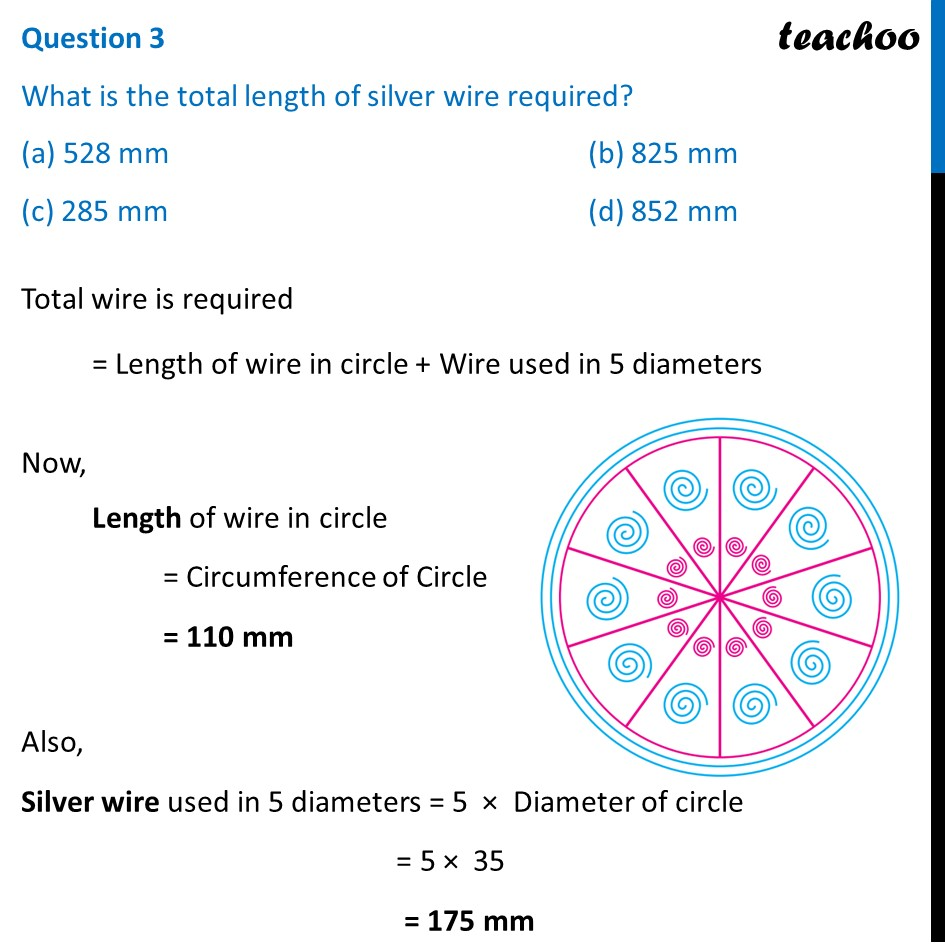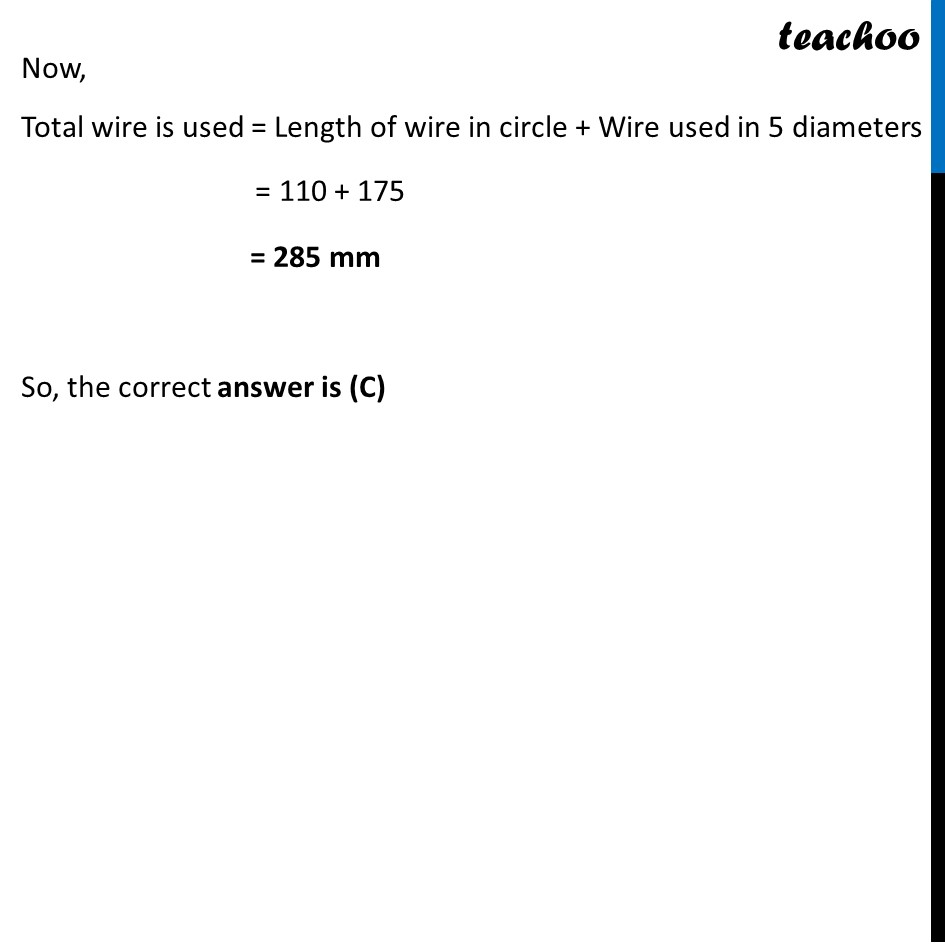## (C) 584/4 mm 2                             (D) 385/4 mm 2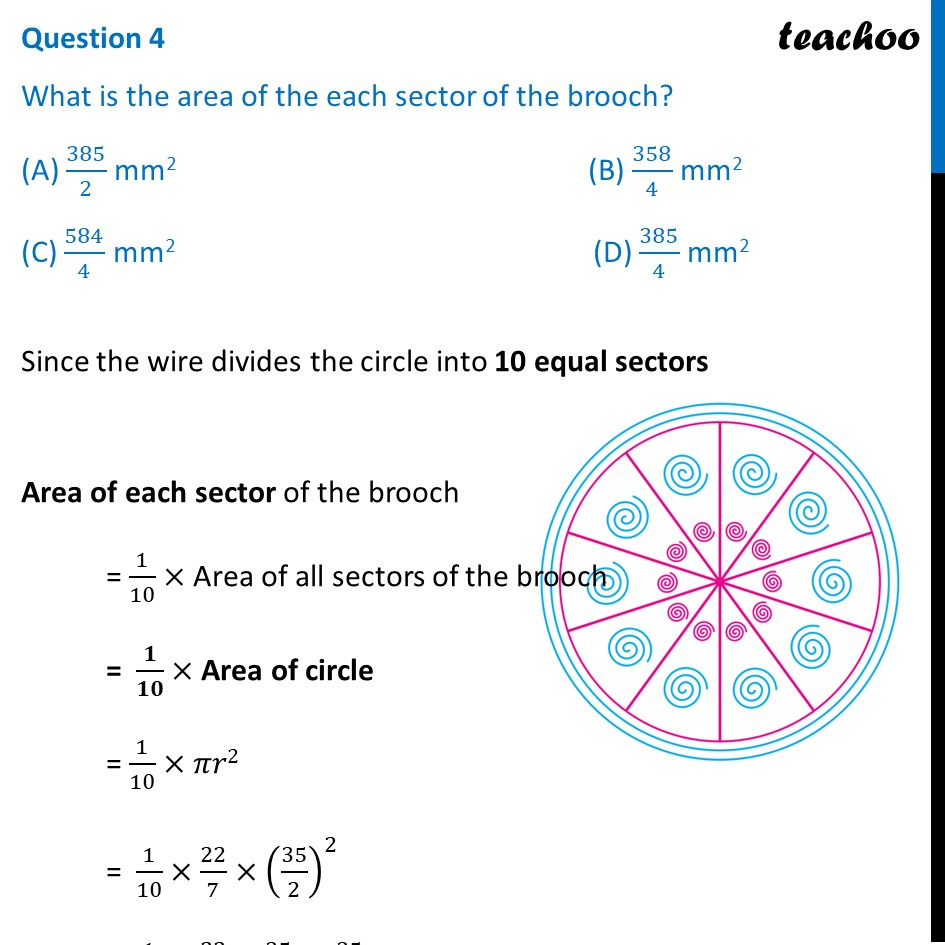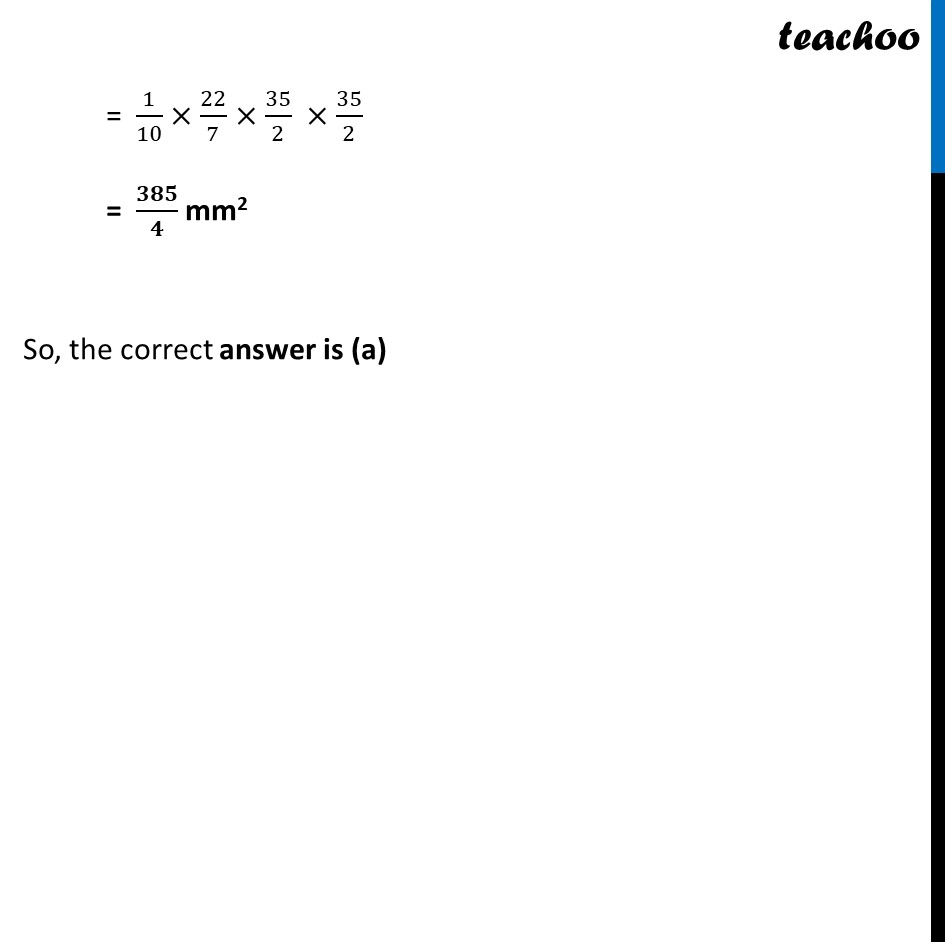## (c) Construction                                     (d) none of these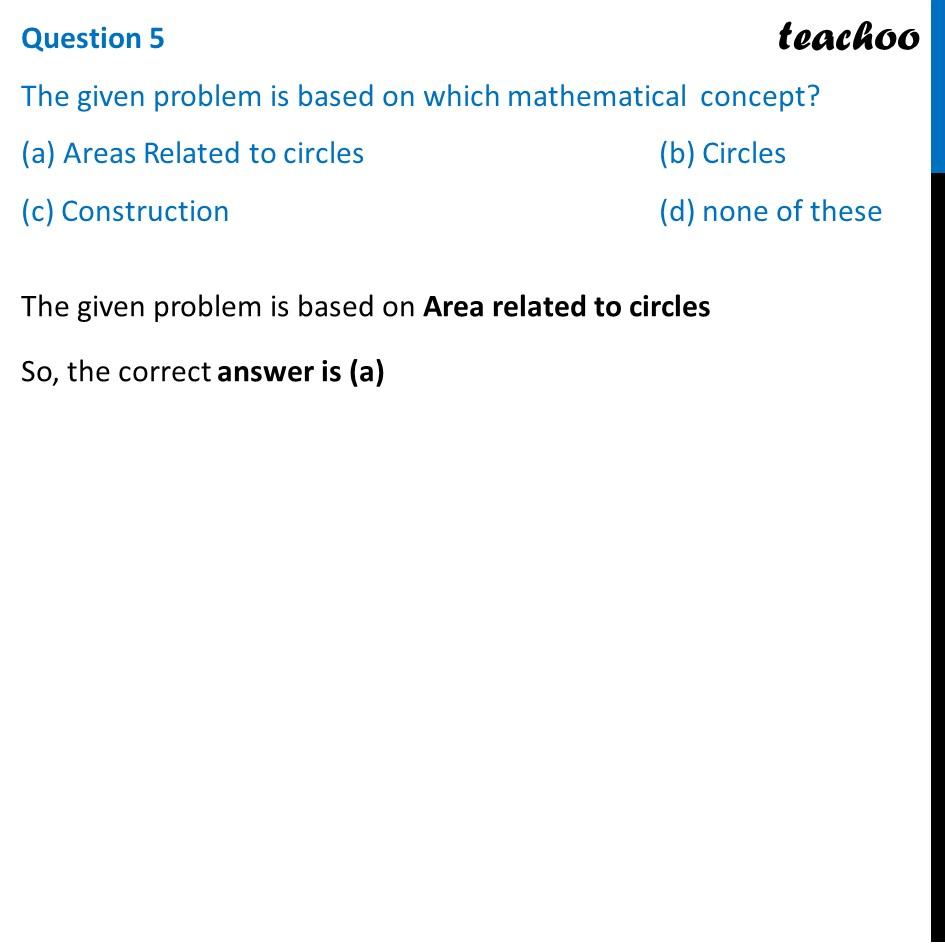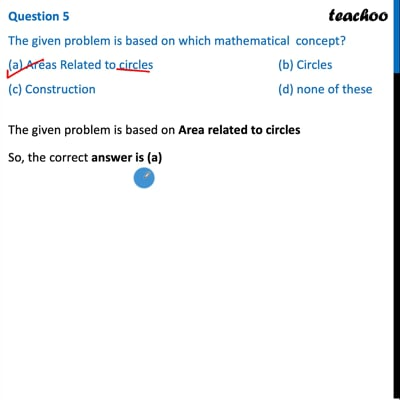This video is only available for Teachoo black users

Introducing your new favourite teacher - Teachoo Black, at only ₹83 per month

### Transcript

Question In a workshop, brooch is made with silver wire in the form of a circle with diameter 35 mm. The wire is also used in making 5 diameters which divide the circle into 10 equal sectors as shown in the given figure. Question 1 What is the radius of the circle? (A) 35/2 mm (B) 5/2 mm (C) 35 mm (C) 10 mm Given Diameter = 35 mm Thus, Radius of circle = Diameter/2 = 𝟑𝟓/𝟐 mm So, the correct answer is (A) Question 2 What is the circumference of the brooch? (a) 100 mm (b) 110 mm (c) 50 mm (d) 10 mm Now, Circumference of brooch = 2𝜋𝑟 = 2×22/7×35/2 = 110 mm So, the correct answer is (B) Question 3 What is the total length of silver wire required? (a) 528 mm (b) 825 mm (c) 285 mm (d) 852 mm vTotal wire is required = Length of wire in circle + Wire used in 5 diameters Now, Length of wire in circle = Circumference of Circle = 110 mm Also, Silver wire used in 5 diameters = 5 × Diameter of circle = 5 × 35 = 175 mm Now, Total wire is used = Length of wire in circle + Wire used in 5 diameters = 110 + 175 = 285 mm So, the correct answer is (C) Question 4 What is the area of the each sector of the brooch? (A) 385/2 mm2 (B) 358/4 mm2 (C) 584/4 mm2 (D) 385/4 mm2 Since the wire divides the circle into 10 equal sectors Area of each sector of the brooch = 1/10× Area of all sectors of the brooch = 𝟏/𝟏𝟎× Area of circle = 1/10× 𝜋𝑟2 = 1/10× 22/7×(35/2)^2 = 1/10× 22/7×35/2 ×35/2 = 𝟑𝟖𝟓/𝟒 mm2 = 1/10× 22/7×35/2 ×35/2 = 𝟑𝟖𝟓/𝟒 mm2 So, the correct answer is (a) Question 5 The given problem is based on which mathematical concept? (a) Areas Related to circles (b) Circles (c) Construction (d) none of these The given problem is based on Area related to circles So, the correct answer is (a)